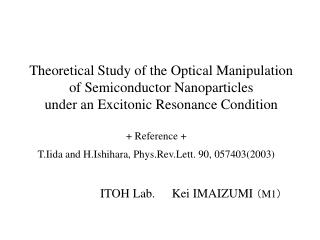DownloadDownload Presentation+ Reference + T.Iida and H.Ishihara, Phys.Rev.Lett. 90, 057403(2003)

# + Reference + T.Iida and H.Ishihara, Phys.Rev.Lett. 90, 057403(2003)

Télécharger la présentation## + Reference + T.Iida and H.Ishihara, Phys.Rev.Lett. 90, 057403(2003)

- - - - - - - - - - - - - - - - - - - - - - - - - - - E N D - - - - - - - - - - - - - - - - - - - - - - - - - - -
##### Presentation Transcript

1. Theoretical Study of the Optical Manipulationof Semiconductor Nanoparticlesunder an Excitonic Resonance Condition + Reference + T.Iida and H.Ishihara, Phys.Rev.Lett. 90, 057403(2003) ITOH Lab.Kei IMAIZUMI （M1）

2. Abstract “Manipulation” means “skillful handling”. Optical manipulation is the technique of handling small objects using mechanical interaction between light and matter. ・Manipulating the semiconductor nanoparticle ・Size selective manipulation Force

3. Contents • Introduction Application, History • Maxwell stress tensor method • Calculation Result The merit of using electronic resonance Heat problem Size selective manipulation • Summary

4. Application Polystyrene bead with a diameter of 1 μm captured by laser Handling of DNA T. T. Perkins, D. E. Smith, S. Chu (1994) Kansai Advanced Research Center http://www-karc.nict.go.jp/ Biology, Chemistry, Material engineering…

5. History of optical manipulation Lorentz derived the equation of motion of a charged particle. Laser was invented. A.Ashkin accelerated micro-size particles by laser. Laser cooling of atoms A.Ashkin demonstrated the trapping of dielectric particle with single focused laser beam. Bose Einstein condensation of atoms 19c 1960 1970 1985 1986 1995 1997 Nobel Prize S.Chu, C.Cohen-Tannoudi, W.D.Phillips 2001 Nobel Prize E.A.Cornell, W.Ketterle, C.E.Wieman

6. Difficulty of manipulating nano-particles Atomic scale r ≪ λ nm μm r ≫ λ difficult ex.) optical tweezer ex.) atom trapping Electronic Resonance • Manipulating the nano-particles • Size selective manipulation

7. Forces Scattering force, Absorbing force Gradient force Resonance The interaction between light and matter increases.

8. Maxwell stress tensor method Lorentz Force volume integral Maxwell Equations < > :time average T : Maxwell’s stress tensor n : normal vector S : Surface of the matter surface integral

9. About the resonance object Incident electric field Eob，Δob constant depending on the object susceptibility resonance P Escat Escat P resonant nonresonant ε：Resonance P：large Escat：large

10. R=100nm R=50nm The merit of using electronic resonance Object CuCl particle hwt = 3.2022 [eV] Greater advantage of resonance about smaller object!

11. The size dependence of its maximum value in the energy range 0 ~ 4 eV. 100nm → No difference 10nm → 104 times lager Handling smaller objectResonance light

12. 〈I〉 〈III〉 R=50nm 〈II〉 Heat problem Absorption Heat problem <I>,<III>・・・Scattering Prevent the heat problem <II>・・・Absorption

13. Size selective manipulation • Quantum size effect -Discrete energy levels -Energy shift depends on the size Energy level

14. Future Image Propagating plane wave Particular particles Application to nanotechnology.

15. Summary • When the size is less than 100nm, the use of electronic resonance has the merit. • The exerted acceleration increases as the size decreases. • The peak position of the force sensitively varies with the size change. Nanoscale size selection nanotechnology

16. Optical tweezer laser R>>λ lens μm Glass sphere (dielectric particle) Force~ pN Force

17. － － － － － + + + + + + Gradient Force Uniform electric field sloping electric field E E cancel each other total force In the sloping electric field, the power works.

18. Quantum size effect Discrete energy levels Atom Semiconductor nanoparticle (quantum dot) Energy shift depends on the size Size change Energy gap change dot size

19. Quantum dot laser Quantum dot laser ・Laser wavelength depends on the dot size. ・Low threshold. ・Stable property at high temperature. Useful property ↑ The same size dots are needed. threshold：しきい値

20. Maxwell stress tensor method Lorentz Force (of N charges) G(t) is the summation of momenta